# Arduino - Servo Motor

In this tutorial, we are going to learn how to use the servo motor with Arduino. In detail, we will learn:

• How servo motor works
• How to program Arduino to control servo motor
• How to program Arduino to control the speed of servo motor
• How to provide the extra power for high-torque servo motors.

## Hardware Required

Or you can buy the following sensor kit:

Please note: These are Amazon affiliate links. If you buy the components through these links, We will get a commission at no extra cost to you. We appreciate it.

Servo motor is a component that can rotate its handle (usually between 0° and 180°). It used to control the angular position of the object.

### Pinout

The servo motor used in this example includes three pins:

• VCC pin: (typically red) needs to be connected to VCC (5V)
• GND pin: (typically black or brown) needs to be connected to GND (0V)
• Signal pin: (typically yellow or orange) receives the PWM control signal from an Arduino's pin.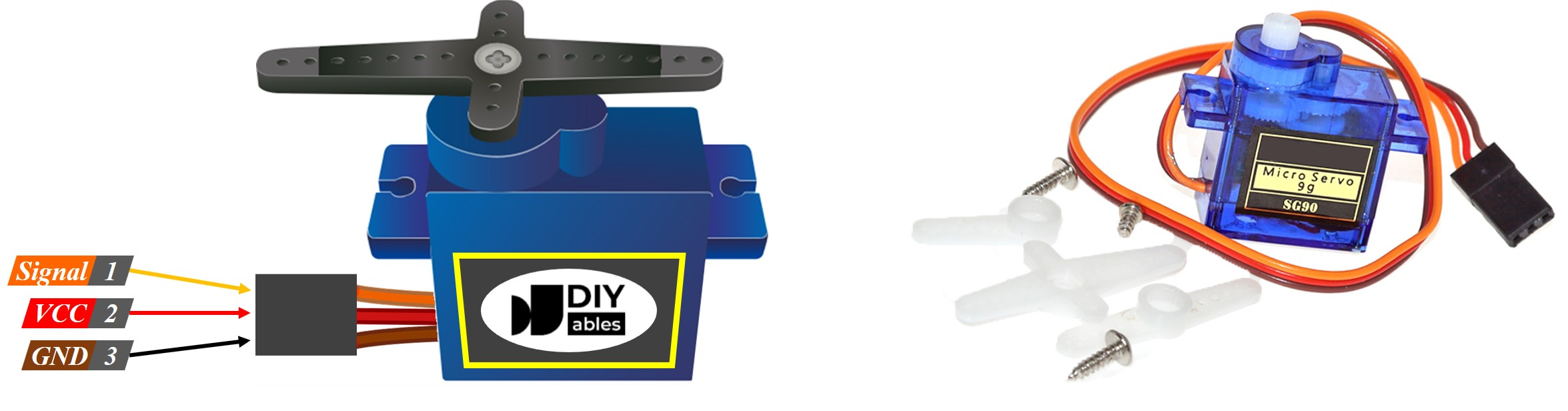### How It Works

This section is the in-depth knowledge. DON'T worry if you don't understand. Ignore this section if it overloads you, and come back in another day. Keep reading the next sections.

After connecting VCC pin and GND pin to 5V and 0V, respectively, we can control the servo motor by generating proper PWM signal to signal pin.

The angle is determined by the width of PWM signal.

Datasheet of the servo motor provides us the following parameters:

• Period of PWM (PERIOD)
• Minimum width of PWM (WIDTH_MAX)
• Maximum width of PWM (WIDTH_MIN)

These parameters are fixed in Arduino Servo library. We do NOT need to know the value of parameters.

The angle is determined as follows:

• If PWM's width = WIDTH_MIN, the servo motor rotates to 0°.
• If PWM's width = WIDTH_MAX, the servo motor rotates to 180°.
• If PWM's width is between WIDTH_MIN and WIDTH_MAX, the servo motor rotates to angle between 0° and 180° in proportion.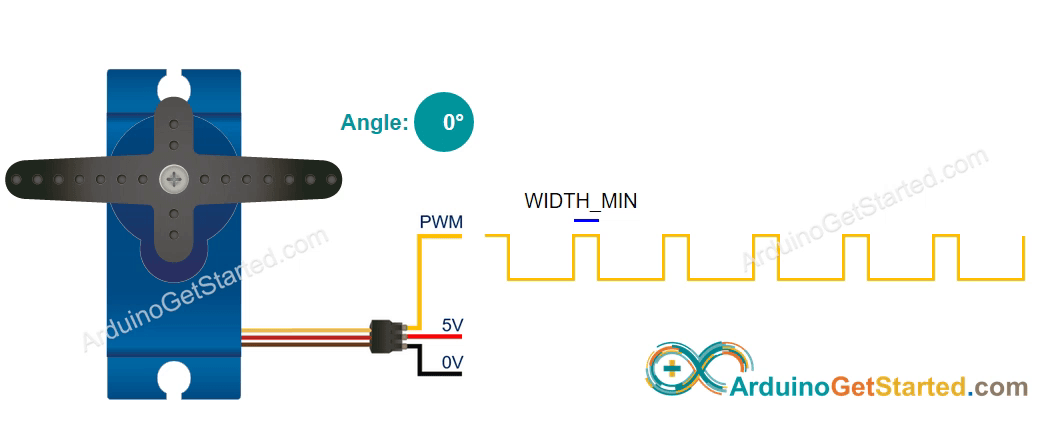### Arduino - Servo Motor

Some of Arduino pins can be programmed to generate PWM signal. We can control the servo motor by connecting the servo motor's signal pin to an Arduino's pin, and programming to generate PWM on the Arduino's pin.

Thanks to Arduino Servo library, controlling servo motor is a piece of cake. We even do NOT need to know how servo motor works. We also do NOT need to know how to generate PWM signal. We JUST need to learn how to use the library.

## Wiring Diagram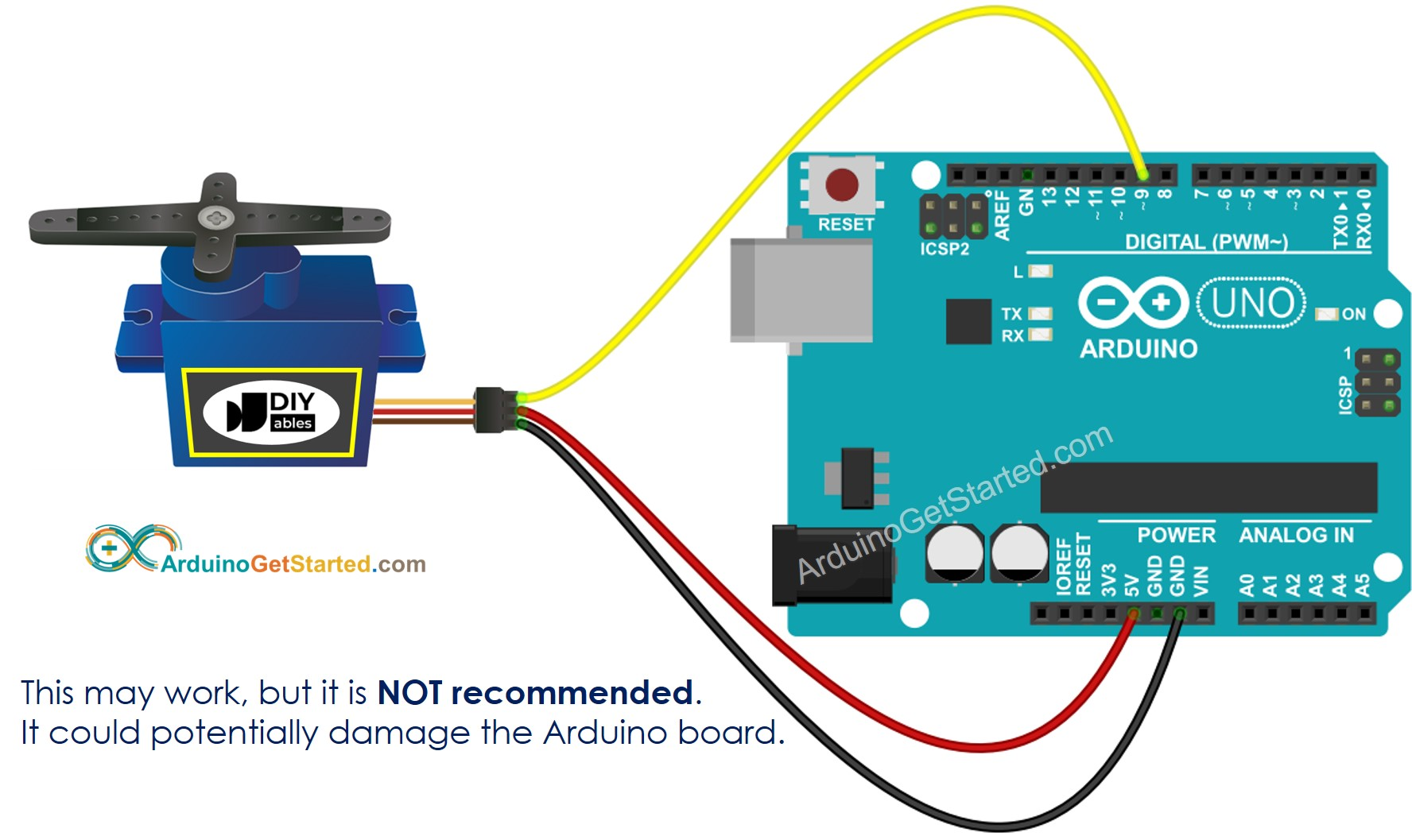This image is created using Fritzing. Click to enlarge image

For the sake of simplicity, the above wiring diagram is used for the testing or learning purposes, and for small-torque servo motor. In practice, we highly recommend using the external power supply for the servo motor. The below wiring diagram shows how to connect servo motor to an external power source.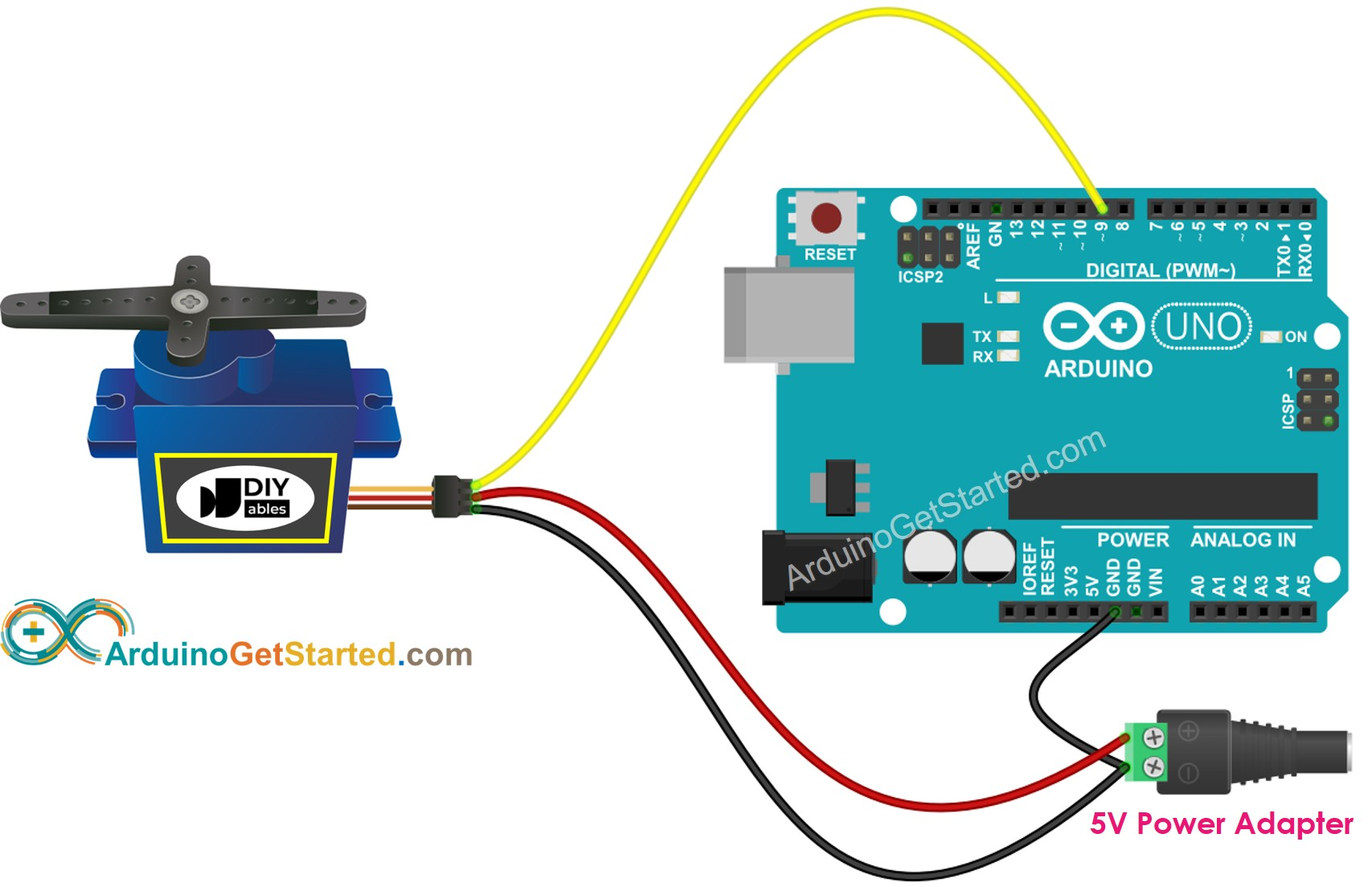This image is created using Fritzing. Click to enlarge image

## How To Program For Servo Motor

• Include the library:
#include <Servo.h>
• Declare a Servo object:
Servo myServo;

If you control more than one servo motors, you just need to declare more Servo objects:

Servo myServo1; Servo myServo2;
• Set the control pin of Arduino, which connects to the signal pin of the servo motor. For example, pin 9:
myServo.attach(9);
• Lastly, rotate the angle of the servo motor to the desired angle. For example, 90°:
myServo.write(90);

## Arduino Code

/* * Created by ArduinoGetStarted.com * * This example code is in the public domain * * Tutorial page: https://arduinogetstarted.com/tutorials/arduino-servo-motor */ #include <Servo.h> Servo servo; // create servo object to control a servo void setup() { servo.attach(9); // attaches the servo on pin 9 to the servo objectư servo.write(0); // rotate slowly servo to 0 degrees immediately } void loop() { for (int pos = 0; pos <= 180; pos += 1) { // rotate slowly from 0 degrees to 180 degrees, one by one degree // in steps of 1 degree servo.write(pos); // control servo to go to position in variable 'pos' delay(10); // waits 10ms for the servo to reach the position } for (int pos = 180; pos >= 0; pos -= 1) { // rotate from 180 degrees to 0 degrees, one by one degree servo.write(pos); // control servo to go to position in variable 'pos' delay(10); // waits 10ms for the servo to reach the position } }

### Quick Steps

• Connect Arduino to PC via USB cable
• Open Arduino IDE, select the right board and port
• Copy the above code and open with Arduino IDE
• Click Upload button on Arduino IDE to upload code to Arduino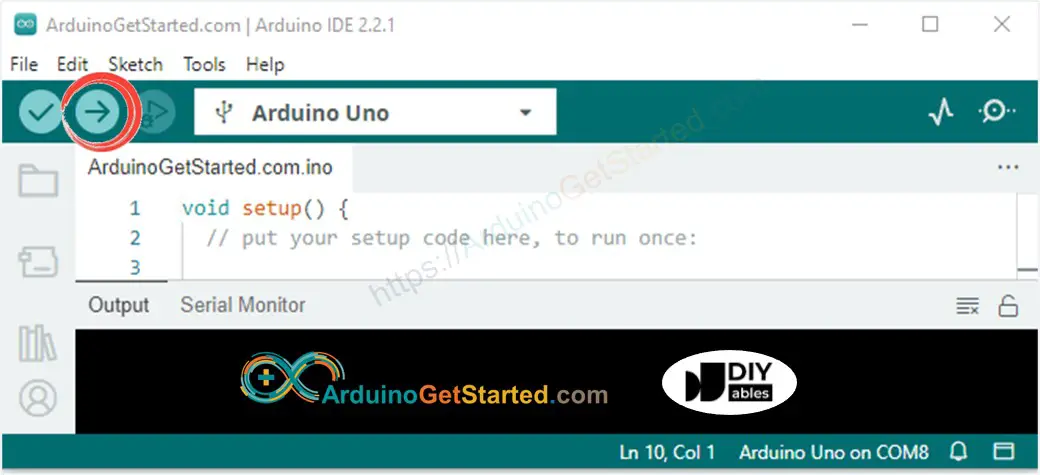• See the result: Servo motor rotates slowly from 0 to 180° and then back rotates slowly from 180 back to 0°

### Code Explanation

Read the line-by-line explanation in comment lines of code!

## How to Control Speed of Servo Motor

By using map() and millis() functions, we can control the speed of servo motor smoothly without blocking other code

/* * Created by ArduinoGetStarted.com * * This example code is in the public domain * * Tutorial page: https://arduinogetstarted.com/tutorials/arduino-servo-motor */ #include <Servo.h> Servo myServo; unsigned long MOVING_TIME = 3000; // moving time is 3 seconds unsigned long moveStartTime; int startAngle = 30; // 30° int stopAngle = 90; // 90° void setup() { myServo.attach(9); moveStartTime = millis(); // start moving // TODO: other code } void loop() { unsigned long progress = millis() - moveStartTime; if (progress <= MOVING_TIME) { long angle = map(progress, 0, MOVING_TIME, startAngle, stopAngle); myServo.write(angle); } // TODO: other code }

## Video Tutorial

We are considering to make the video tutorials. If you think the video tutorials are essential, please subscribe to our YouTube channel to give us motivation for making the videos.

## Challenge Yourself

Use the servo motor to do one of the following projects:

• The Servo library supports up to 12 motors on Arduino UNO and 48 on the Arduino Mega.
• Power from 5V pin of Arduino maybe NOT enough for servo motor in one of the following cases:
• Using a high-torque servo motor, which can carry a high-weight thing.
• Using many servo motors.

In these cases, we may need to provide an extra power source for servo motors.This image is created using Fritzing. Click to enlarge image

As we can see in the above diagram, the VCC pin of servo motor doest NOT connect to the 5V pin of Arduino. It connects to the positive pin of an extra power source.